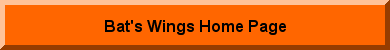AP Chapter 7 – Example 1 – page 70 -- Prediction of High and Low Tide  (the answers are printed in your book - page 71)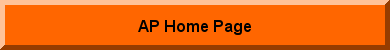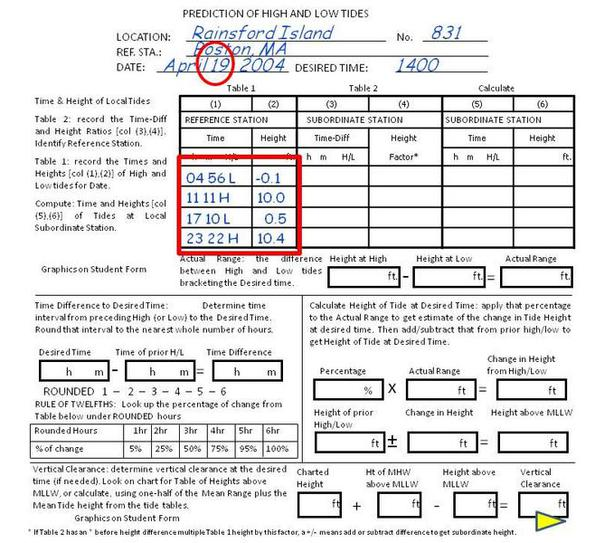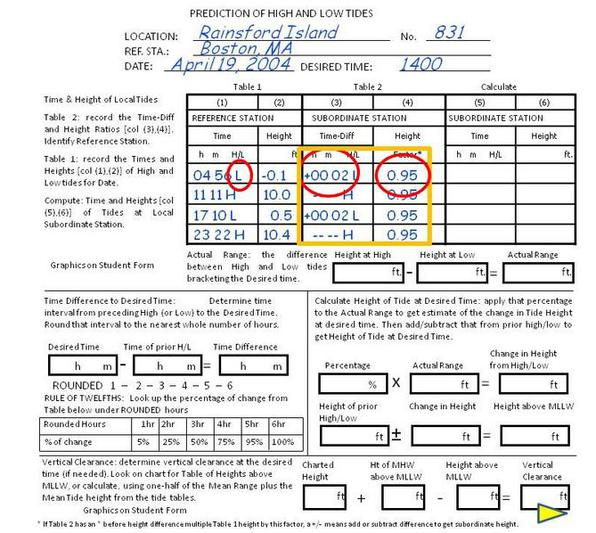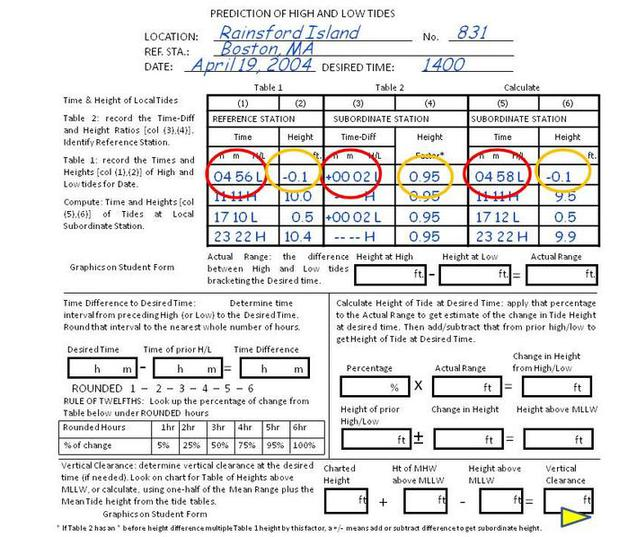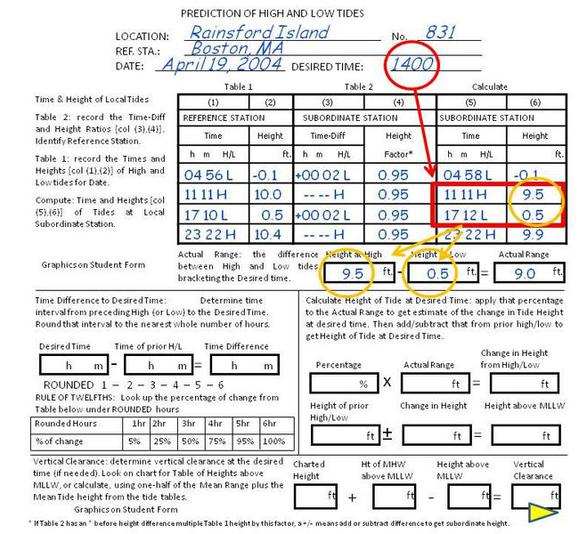Location:Rainsford Island – Nantasket
Date:Apr 19, 2004
Desired Time:1400 EST

Need:
Prediction of High and Low Tides (form)
Tide Table (Newport, Rhode Island) April 2004
Subordinate Station (Table 2) (Station # 831)First, fill in the top of the form shown in figure 7-4.  (You can copy these forms.)

Don't forget, all the tide tables are in Standard time, not Daylight Savings.  Our desired time is 1400 EST.

Next, go to the Tide Tables (Table 1) for your Reference Station, Boston, on the Day of interest (April 19, 2004).  (See figure 7-2 in the book, page 68.)  Fill in the times, and height, of each tide that day.  Also, label the time "H" for High or "L" for Low - this helps prevent errors later.

These values are outlined in Red on the form.Next, go to the Subordinate Table (Table 2) for  the entries for Rainsford Island (Fig 7-3, p 68).  Rainsford is station number 831.  Each Subordinate Station has one set of figures, which are applied to the Reference station's daily values.

Fill in the Subordinate Station values (Outlined in Gold).  These are differences in time and height for each tide.

Since the first tide for Boston on the 19th is a Low tide, fill in the time difference (+ 2 min) and the height ratio (.95) on the first line.  You can see it's easy to make an error, writing the subordinate station differences for the High tide in the line for the low tide, or vise versa

At Rainsford Island, low tide occurs 2 minutes after low tide at Boston, and is 95% of the value of the low tide at Boston.

High tide is the same time as Boston, also at 95% of Boston's value.
Next, calculate the time and heights of the high and low tide at the Subordinate Station.

In this example, the reference station has a low of 0456, a difference of +2 min, for a low tide time of 0458.  The level of tide is -0.1 ft at the reference station, multiplied by the ratio of .95, which rounds off to -.1 ft low tide at Rainsford.

Now that you have the high and low tides for Rainsford, determine the tide at the time of interest (1400 hrs).

Bracket the time of interest (red box) and determine the tidal range from High to low (or low to high), which calculates to 9.0 ft.

Then, you will calculate, based on the "Rule of Twelfths", how much the tide has risen or fallen by 1400 hrs.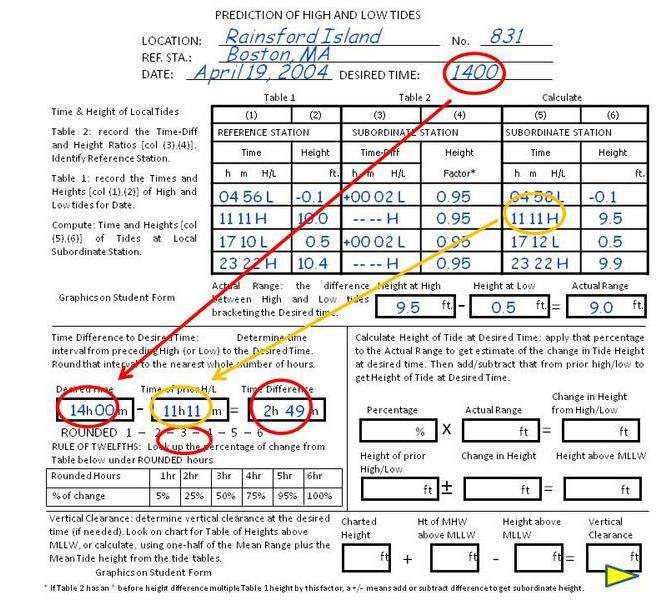Determine where your time falls between High and Low tide.

Your time of interest is 1400.

High is at 1111.  The next low is at 1712.  The range is 9.0 feet.  Therefore, the tide at 1400 will be somewhere between the levels at 1111 and 1712.

Subtract the time of the preceding high/low (1111) from the desired time (1400).  This shows that 1400 occurs 2h and 49m after high tide.

​To simplify the calcuation, this rounds out to 3 hours, so the tide has dropped 50% from High to the time of interest.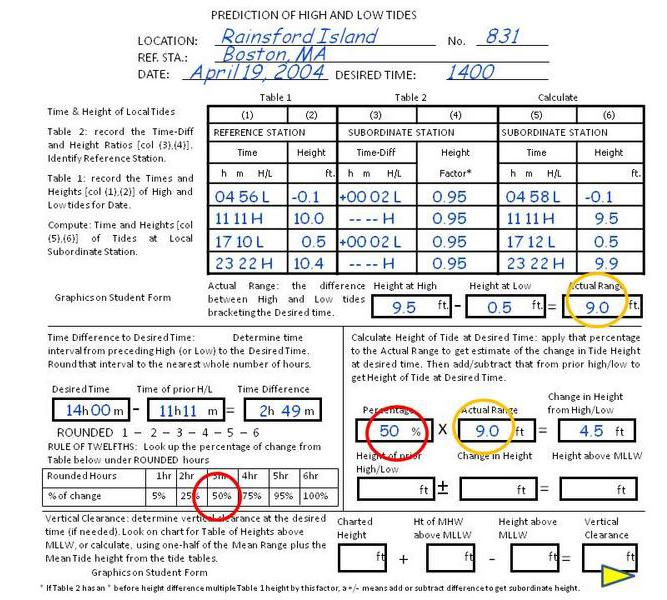Here, you simply multiply 50% times the 9.0 ft tidal range, to see the tide has dropped 4.5 ft.

You know it has dropped, because the earlier tide (1111) was a high, and the next tide (1712) is a low.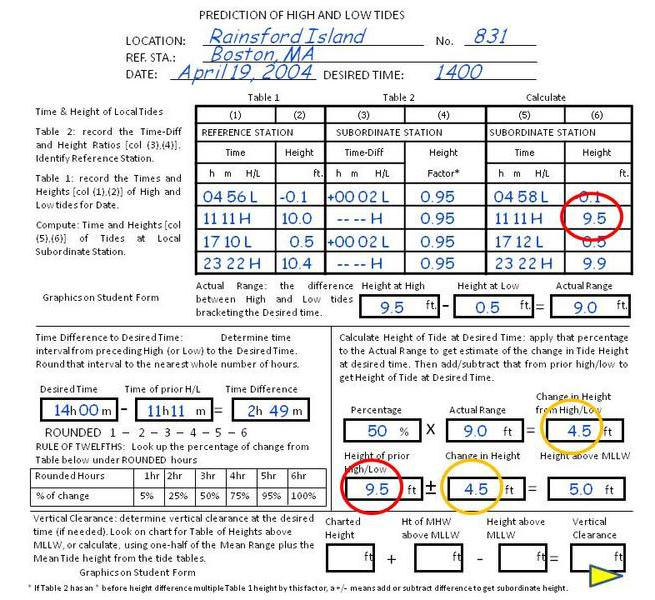Finally, you take the height of the preceding high or low tide (it was a high), and add or subtract the change in the tide, to get the actual tide height of the time of interest, which is 1400 hrs.

In this case, you know to subtract the change, because the tide at 1111 was a high, and  the tide is going down.

If the time of interest was between 0458 and 1111, you know the tide is going up, so you would ADD the change in height to the height at 0458.

In our example, the tide at 1400 is calculated to be 5.0 feet above MLLW.